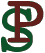Lagrange Applet

It is easy to have the Lagrange Applet simulate your own dynamical system. For each dynamical system, you have to define a subclass of the class `MechSystem`. The source code of the Lagrange Applet is in the file Lagrange.java. This is where the individual mechanical systems, such as SpringPendulum, are defined. You can edit this file to implement other mechanical systems. This document describes how to write the code to do so.

Fortunately, the pre-defined class `MechSystem` already implements all the gory details of Lagrangian mechanics and the actual simulation. To define your own mechanical system, you only need to provide information that describes the physics of the particular system that you want to simulate. There are three groups of methods that need to be defined:

1. Methods that return information about the mechanical system, such as the number and names of its parameters and coordinates.
2. Methods that calculate the kinetic and potential energy for the system.
3. Methods that define how the state of the system is to be drawn.
Optionally, you may define a fourth group of methods that define how to handle mouse events.

In the methods that you will define, all the calculations are done in actual physical units, such as meters and kilograms, and not in computer units, such as pixels. The relevant conversions to screen coordinates etc. are automatically handeled by the superclass `MechSystem`. This way, you only need to worry about the physical properties of the mechanical system you are defining.

### Example: A Spring Pendulum

As an example, we will examine how the class SpringPendulum is defined. A spring pendulum is a double pendulum, where the inner leg of the pendulum is a spring, like this: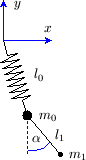Let m0 and m1 be the inner and outer mass, respectively. Let l0 and l1 be the length of the inner and outer leg, where l0 is the length of the spring in its relaxed state. Let k0 be the spring constant. Masses are measured in kilograms, lengths in meters, and spring constants in Newton/meter. Also, let g=9.81m/s2 be the gravitational constant. The system has three coordinates: x and y are the coordinates of the first mass, measured in meters in a coordinate system that has the pivot point as its origin. Alpha is the angle of the outer leg, measured in radians counterclockwise from south. We number the coordinates: q0=x, q1=y, q2=alpha.

The kinetic and potential energy of the spring pendulum are given by: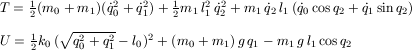### How to define the SpringPendulum class

We will now see how to translate this physical information into a Java class. The class SpringPendulum is defined as follows:
```class SpringPendulum extends MechSystem {
```

#### Instance variables and general methods

First, you may declare any internal variables you are going to use. Typically there will be one variable for each parameter (such as masses, etc.). In this case, we also declare two integer variables that will hold the radii of the dots that will represent the two masses.
```  private double m0, m1, l0, l1, k0, g; // physical parameters
```
Next, we need to define three methods that provide general information about the system. The method `getType()` simply returns the name of this class.
```  String getType() {
return "SpringPendulum";
}
```
The method `getParamInfo()` returns information about the physical parameters. In the case of the spring pendulum, there are six parameters. The method returns an array of objects of type `Parameter`. Each entry describes one parameter, by giving its name, brief description, physical units, and default value.
```  Parameter[] getParamInfo() {
Parameter[] paraminfo = {
new Parameter("m0", "inner mass", "kg", 2),
new Parameter("m1", "outer mass", "kg", 1),
new Parameter("l0", "inner length", "m", 6),
new Parameter("l1", "outer length", "m", 4),
new Parameter("k0", "spring constant", "N/m", 60),
new Parameter("g", "gravity", "m/s^2", 9.81),
};
return paraminfo;
}
```
The method `getCoordInfo()` returns information about the coordinates. In this case, there are three coordinates: x, y, and alpha. The method returns an array of objects of type `Coordinate`. Each entry describes one coordinate, by giving its name, a brief description, physical units, default initial value, default initial value of the derivative, and the period of this coordinate. For instance, the period of the coordinate alpha is 2pi. The coordinates x and y are not periodic, so the period is set to 0.
```  Coordinate[] getCoordInfo() {
Coordinate[] coordinfo = {
new Coordinate("x", "coordinate of inner mass, right of pivot", "m",
2.2, 0, 0),
new Coordinate("y", "coordinate of inner mass, above pivot", "m",
3, 0, 0),
new Coordinate("alpha",
"angle of outer leg, counterclockwise from south",
"radians", 4, 0, 2*Math.PI),
};
return coordinfo;
}
```
The method `setphysics()` is used to set the internal variables that depend on physical parameters, such as m0, m1, etc. It is called during initialization, and also each time one of the parameters changes. The values of the parameters are passed in a `double` array `param`. The parameters appear in this array in the order in which `getParamInfo()` declares them. This is also the place to set any other internal variables that depend on the parameters, such as the radii of the two masses in this example.
```  void setphysics(double[] param) {
m0=param;
m1=param;
l0=param;
l1=param;
k0=param;
g =param;
}
```

#### Methods that define the kinetic and potential energy

We now turn to the methods that compute the kinetic and potential energy. These are simply the translations of the respective physical formulas. The current values of the coordinates, and their current first derivatives, are passed in the `double` arrays `q`, respectively, `qp`. The method `sq(double)`, defined in the class `MechSystem`, may be used for squaring a value.

The simulation of the Lagrange Applet has preservation of energy built-in, so that the system does not spin out of control as easily in the face of numerical errors. For that reason, neither the potential nor the kinetic energy are allowed to have an explicit time dependency. Also, the algorithm used to adjust the total energy uses the fact that the kinetic energy depends quadratically on the first derivatives of the coordinates, and it is likely to fail if the kinetic energy does not have this property. (However, forced preservation of energy can also be turned off).

```  // kinetic energy
double T(double q[], double qp[]) {
return (m0+m1)/2 * (sq(qp)+sq(qp))
+    m1/2 * sq(l1)*sq(qp)
+    m1 * qp*l1*(qp*Math.cos(q)+qp*Math.sin(q));
}

// potential energy
double U(double q[]) {
return k0/2 * sq(Math.sqrt(sq(q)+sq(q))-l0)
+ (m0+m1)*g * q
- m1*g * l1*Math.cos(q);
}
```
The superclass `MechSystem` defines three methods `diffq(int i, double q[], double qp[])`, ```diffpp(int i, int j, double q[], double qp[])```, and ```diffqp(int i, int j, double q[], double qp[])``` that use a numerical method to calculate the respective partial derivatives (where L = T - U):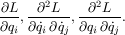If you wish, you may override these with methods that calculate the relevant partial derivatives using an exact formula; this will slightly enhance performance.

#### Methods that define how the system is drawn

Next, we need to define two methods that define how the mechanical system is to be drawn. The method `drawsystem()` draws the current state. For this purpose, it may call the methods `drawSpring()`, `drawLine()`, `drawMass()`, `drawCircle()`, and `recordTrace()`, all of which are provided by the superclass `MechSystem`. These methods expect physical coordinates (in meters); conversions to screen coordinates are taken care of by the superclass.

The `recordtrace` method must be invoked to draw a trace segment; this is drawn on the background `bg` as well as on the foreground `og`.

The method `drawsystem()` may also invoke arbitrary drawing methods on the Graphics object `og`; however, in this case, the coordinates must be converted explicitly, for instance ```og.drawRectangle(c.x(left), c.y(top), c.dx(right-left), c.dy(bot-top))```. The methods `c.x()` and `c.y()` convert physical coordinates to screen coordinates, and the methods `c.dx()` and `c.dy()` convert differences of physical coordinates to differences of screen coordinates. Note that in the physical coordinate system, the y-axis points upwards. Before invoking a drawing method of `og`, the correct color must be set with `og.setColor(Global.fgcolor)`.

```  void drawsystem(Graphics og, Graphics bg) {
double x0 =  q;
double y0 =  q;
double x1 =  x0+l1*Math.sin(q);
double y1 =  y0-l1*Math.cos(q);

recordTrace(bg,og,x1,y1);

drawSpring(og,0,0,x0,y0,15,0.2);
drawLine(og,x0,y0,x1,y1);
}
```
The method `bounds()` is used to determine the size of the image that `drawsystem()` is going to draw. It returns a rectangle of physical coordinates that represents upper and lower bounds on the coordinates used by the drawing methods in `drawsystem()`. It is important that this rectangle is big enough to draw not just the current state, but also future states that may arise as the system evolves. Conservation of energy may be assumed for this purpose; should the energy change, the method `bounds()` is called again. The method `E()` can be called to calculate the current total energy of the system. The result should be returned with the statement ```return new DoubleRectangle(left,bot,right,top).enlarge(.05)```, where the `enlarge()` method on a rectangle adds a little space around the edges.
```  DoubleRectangle bounds() {
double l=l1+l0+
Math.sqrt(sq((m0+m1)*g/k0)+2*l0*(m0+m1)*g/k0+2*m1*g/k0+2*E()/k0);
double equi=(m0+m1)*g/k0;

return new DoubleRectangle(-l,-equi-l,l,-equi+l).enlarge(.05);
}
```

#### Mapping mouse events (optional)

Finally, the method `mouseMap()` maps (x,y)-coordinates to internal coordinates for the purpose of mouse action. It should contain a sequence of statements of the form `map(i,v)`, which means set the ith coordinate to the value v. In the case of our spring pendulum, the definition of `mouseMap()` is simply this:
```  void mouseMap(double x, double y) {
map(0, x);
map(1, y);
}
```
This means that when the mouse is clicked on coordinates (x,y), then the coordinate q0 of the dynamical systems is fixed to x and the coordinate q1 is fixed to y. If we had some other mechanical system, where the coordinate q0 denoted some angle, measured counterclockwise from south, we would define
```  void mouseMap(double x, double y) {
map(0, Math.atan2(x,-y));
}
```
The default implementation of `mouseMap` does nothing; so if it is not overridden, then mouse clicks and drags will have no effect. This finishes the definition of the class `SpringPendulum`.

### Other modifications to Lagrange.java

Finally, you must modify the code of the main class Lagrange so that it can recognize the mechanical system you have just defined. You must add code in the following two places:
```    (new DoublePendulum()).appendInfoVector(infov);
(new DoubleSpring()).appendInfoVector(infov);
(new SpringPendulum()).appendInfoVector(infov);
// *** add your own system here ***
...

if (type.equals("doublependulum")) {
mechsys = new DoublePendulum();
} else if (type.equals("springpendulum")) {
mechsys = new SpringPendulum();
} else if (type.equals("doublespring")) {
mechsys = new DoubleSpring();
// *** add your own system here ***
```
This is it. Have fun designing your own mechanical systems!

Back to main Lagrange page.
Back to Peter Selinger's Homepage: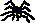Peter Selinger / Department of Mathematics and Statistics / Dalhousie University
selinger@mathstat.dal.ca / PGP key
Updated June 3, 2001